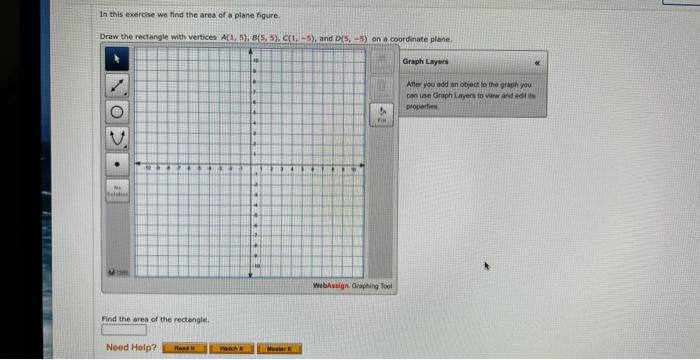Home / Expert Answers / Algebra / in-this-exercise-we-find-the-area-of-a-plane-figure-draw-the-rectangle-with-vertices-nbsp-a-1-nbsp-pa507

# (Solved): In this exercise we find the area of a plane figure.Draw the rectangle with vertices A(1,  ...

In this exercise we find the area of a plane figure.

Draw the rectangle with vertices
A(15), B(55), C(1?5), and D(5?5)
on a coordinate plane.Find the area of the rectangle.

In this exercise we find the area of a plane figure. Draw the rectangle with vervices \( A[1,5, B(5,5), C(1,-5) \), and \( D(5,-5) \) on a coorbliate plane, Graph Laytis Ane you add an objock to the graph you caniabe Graph Lajen fo wirk ard nod iss properties. Find the area of the rectengle.

We have an Answer from Expert